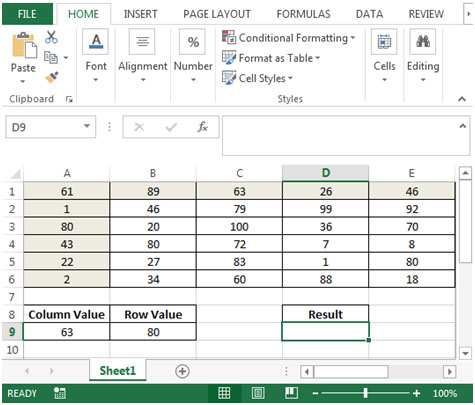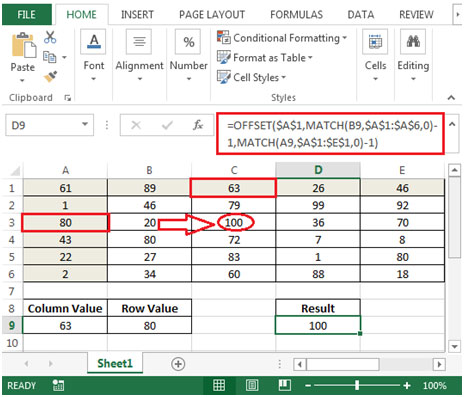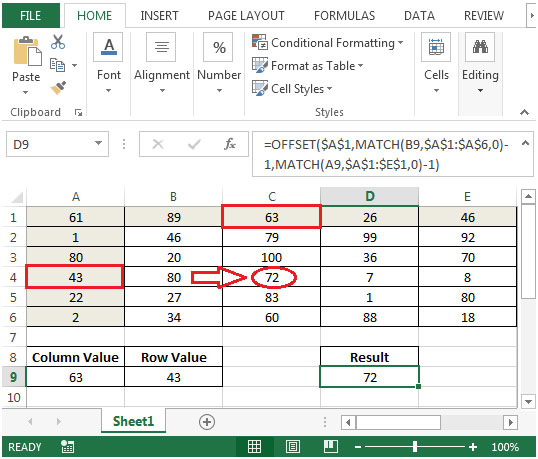Retrieving a Value from a Reference Grid, Using Indexes Listed in another Range

In this article we will learn how to find a value from a reference grid while using indexes list, we can use a combination of "OFFSET" & "MATCH" functions to get the output in Microsoft Excel 2010.
OFFSET: Returns areference to a range that is a given number of rows and columns from a given reference.

Syntax: =OFFSET(reference,rows,cols,height,width)

reference: It is a reference to a cell or range of cells from which the offset will be applied.

rows: The number of rows up or down that you want to apply as the offset to the range.

cols: The number of columns left or right that you want to apply as the offset to the range.

height: This is optional. It is the number of rows that you want the returned reference to be.

width: This is optional. It is the number of columns that you want the returned reference to be.
MATCH function searches for a specified item in a selected range of cells, and then returns the relative position of that item in the range.

Syntax =MATCH(lookup_value,lookup_array,match_type)

lookup_value: The value you want to look for

lookup_array: The table of data contains information from which you want to return the output.

match_type: 1,0 and -1 are three options.

1(Default): It will find the largest value in the range. List must be sorted in ascending order.

0: It will find an exact match

-1: It will find the smallest value in the range. List must be sorted in descending order.
Let us take an example:

We have a list of some random numbers in range A1:E6.Cell A9 contains value from column range i.e. A1:E1. Cell B9 contains value from row range i.e. A1:A6. We need a formula to find the matching value from both column & row.• In cell D9, the formula would be
• =OFFSET(\$A\$1,MATCH(B9,\$A\$1:\$A\$6,0)-1,MATCH(A9,\$A\$1:\$E\$1,0)-1)
• Press enter on your keyboard.
• The function will return the result 100 (cell C3) Column C is 63 and the value of 3rd Row is 80.• If we change the row value in cell B9 from 80 to 43, then the result would be calculated automatically.Terms and Conditions of use

The applications/code on this site are distributed as is and without warranties or liability. In no event shall the owner of the copyrights, or the authors of the applications/code be liable for any loss of profit, any problems or any damage resulting from the use or evaluation of the applications/code.